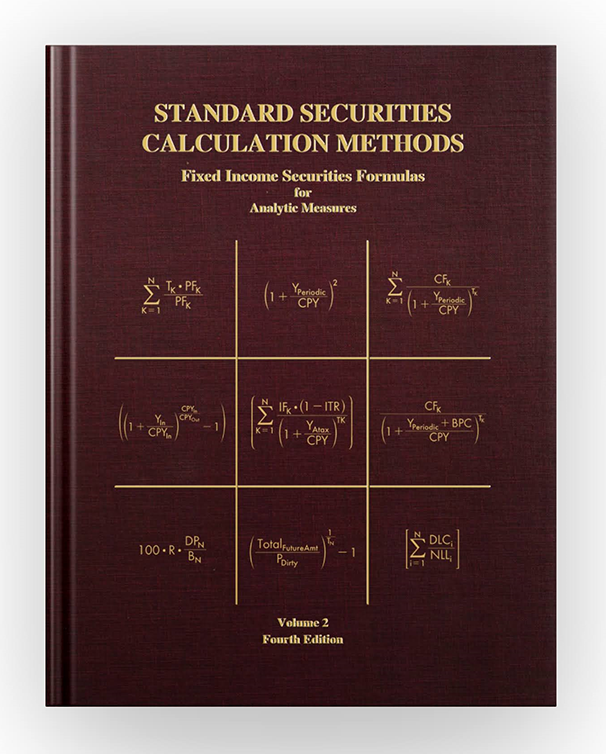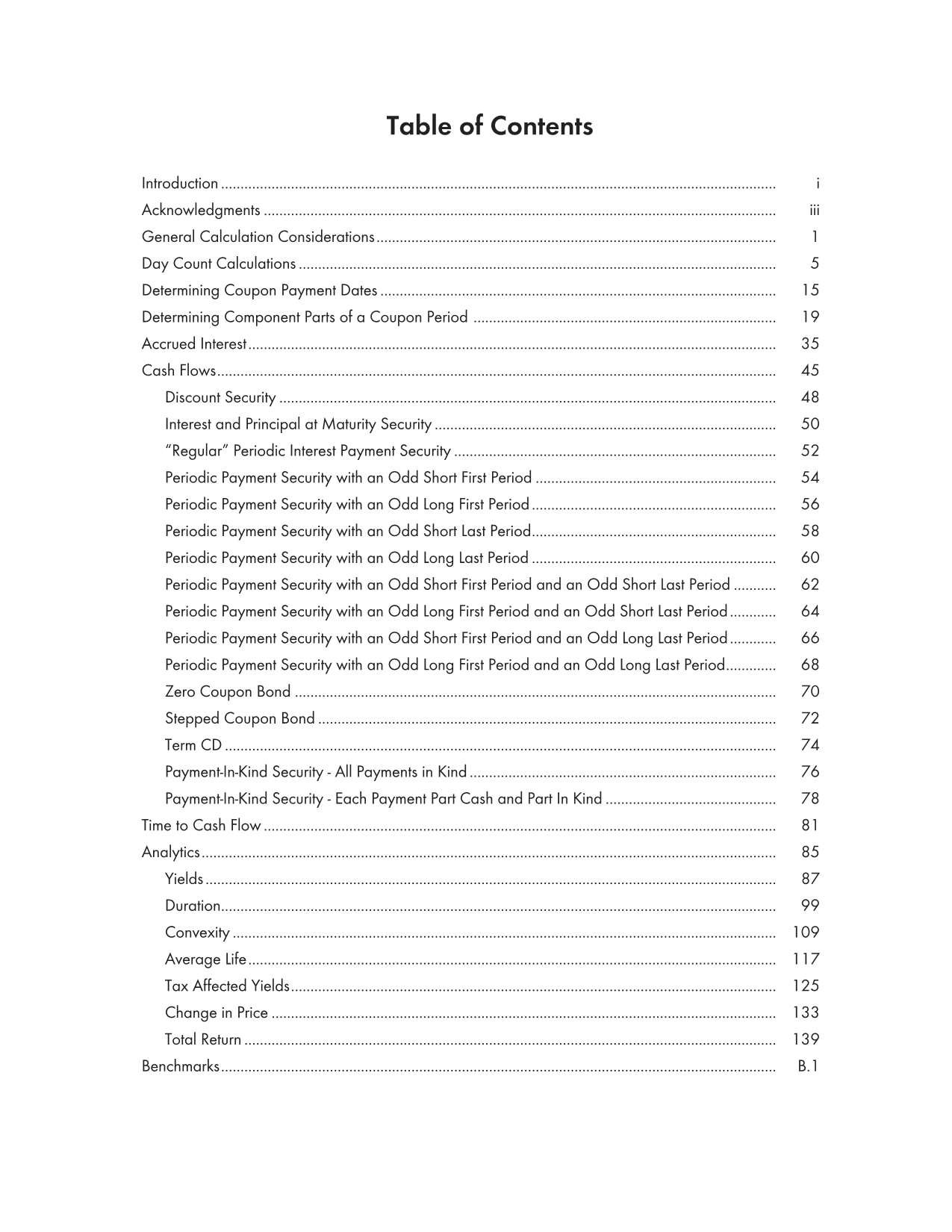Standard Securities Calculation Methods

Fixed Income# Fixed Income Securities FormulasforAnalytic Measures

This Fourth Edition of Volume 2 is an update of the 1996 edition, plus additional new material. Volume 2 of the Standard Securities Calculation Methods books presents a compilation of formulas for the calculation of analytics for the current diversity of fixed income securities.

The formulas and methods presented here provide for the uniform calculation and application of these analytics across fixed income security types.

This book is organized in nine basic sections: (1) General Calculation Considerations, (2) Determining Coupon Payment Dates, (3) Determining Component Parts of a Coupon Period, (4) Day Count Calculations, (5) Accrued Interest, (6) Cash Flows, (7) Time to Cash Flow, (8) Analytics, and (9) Benchmarks.

The general approach for the computations is to first determine the future cash flows for a particular security; then, any of the formulas in the Analytics section (yields, duration, convexity, average life, tax affected yields, change in price, and total return) can be calculated. In this way, diverse securities with known cash flows can be compared on an equal footing, without considering their possibly different day count methodologies, coupon frequencies, simple interest formulas, ex-dividends, etc.

The yield formulas presented in this volume are not intended to replace the yield formulas presented in Volume 1. Volume 1’s formulas are the accepted industry conventions, and are used for trading and settlement purposes; the yield formulas in this volume provide a standardized method for comparing any fixed income security to any other, on a yield basis.

New and updated in the Fourth Edition:

• Cash flow generation formulas have been added for Payment in Kind (PIK) securities.
• The 30/360 Day Count Basis methodology has been adjusted to consider coupon payment cycles (End-of-Month rule).
• A section detailing how to calculate the component parts of a period has been added.
• All of the calculation examples and Benchmarks have been updated to reflect current generally accepted practices and calculation rules.